# 635 Results

View
Selected filters:
• CCSS Math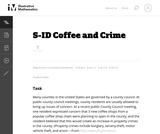Unrestricted Use
CC BY
Rating

This task addresses many standards regarding the description and analysis of bivariate quantitative data, including regression and correlation. Students should recognize that the pattern shown is one of a strong, positive, linear association, and thus a correlation coefficient value near +1 is plausible. Students should also be able to interpret the slope of the least-squares line as an estimated increase in y per unit change in x (and thus for a 3 unit increase in x, students should expect an estimated increase in y that equals 3 times the model's slope value).

Subject:
Mathematics
Material Type:
Activity/Lab
Provider:
Illustrative Mathematics
Provider Set:
Illustrative Mathematics
Author:
Illustrative Mathematics
02/19/2013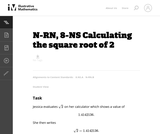Unrestricted Use
CC BY
Rating

This task is intended for instructional purposes so that students can become familiar and confident with using a calculator and understanding what it can and cannot do. This task gives an opportunity to work on the notion of place value (in parts (b) and (c)) and also to understand part of an argument for why the square root of two is not a rational number.

Subject:
Mathematics
Material Type:
Activity/Lab
Provider:
Illustrative Mathematics
Provider Set:
Illustrative Mathematics
Author:
Illustrative Mathematics
11/13/2012Unrestricted Use
CC BY
Rating

The given solutions for this task involve the creation and solving of a system of two equations and two unknowns, with the caveat that the context of the problem implies that we are interested only in non-negative integer solutions. Indeed, in the first solution, we must also restrict our attention to the case that one of the variables is further even.

Subject:
Mathematics
Algebra
Material Type:
Activity/Lab
Provider:
Illustrative Mathematics
Provider Set:
Illustrative Mathematics
Author:
Illustrative Mathematics
01/18/2013Unrestricted Use
CC BY
Rating

This task examines the ways in which the plane can be covered by regular polygons in a very strict arrangement called a regular tessellation. These tessellations are studied here using algebra, which enters the picture via the formula for the measure of the interior angles of a regular polygon (which should therefore be introduced or reviewed before beginning the task). The goal of the task is to use algebra in order to understand which tessellations of the plane with regular polygons are possible.

Subject:
Mathematics
Algebra
Geometry
Material Type:
Activity/Lab
Provider:
Illustrative Mathematics
Provider Set:
Illustrative Mathematics
Author:
Illustrative Mathematics
01/21/2013Unrestricted Use
CC BY
Rating

This task provides a simple but interesting and realistic context in which students are led to set up a rational equation (and a rational inequality) in one variable, and then solve that equation/inequality for an unknown variable.

Subject:
Mathematics
Algebra
Material Type:
Activity/Lab
Provider:
Illustrative Mathematics
Provider Set:
Illustrative Mathematics
Author:
Illustrative Mathematics
12/15/2012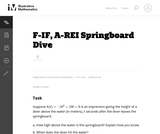Unrestricted Use
CC BY
Rating

The problem presents a context where a quadratic function arises. Careful analysis, including graphing, of the function is closely related to the context. The student will gain valuable experience applying the quadratic formula and the exercise also gives a possible implementation of completing the square.

Subject:
Mathematics
Functions
Material Type:
Activity/Lab
Provider:
Illustrative Mathematics
Provider Set:
Illustrative Mathematics
Author:
Illustrative Mathematics
05/01/2012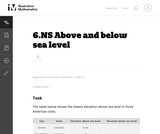Unrestricted Use
CC BY
Rating

The purpose of this task is to help students interpret signed numbers in a context as a magnitude and a direction and to make sense of the absolute value of a signed number as its magnitude.

Subject:
Mathematics
Material Type:
Activity/Lab
Provider:
Illustrative Mathematics
Provider Set:
Illustrative Mathematics
Author:
Illustrative Mathematics
05/01/2012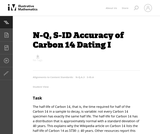Unrestricted Use
CC BY
Rating

This task examines, from a mathematical and statistical point of view, how scientists measure the age of organic materials by measuring the ratio of Carbon 14 to Carbon 12. The focus here is on the statistical nature of such dating. This task addresses a very important issue about precision in reporting and understanding statements in a realistic scientific context.

Subject:
Mathematics
Material Type:
Activity/Lab
Provider:
Illustrative Mathematics
Provider Set:
Illustrative Mathematics
Author:
Illustrative Mathematics
09/13/2012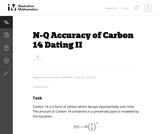Unrestricted Use
CC BY
Rating

This task is a refinement of ``Carbon 14 dating'' which focuses on accuracy. Because radioactive decay is an atomic process modeled by the laws of quantum mechanics, it is not possible to know with certainty when half of a given quantity of Carbon 14 atoms will decay. This type of question is very important in science and it also provides an opportunity to study the very subtle question of how errors behave when applying a function: in some cases the errors can be magnified while in others they are lessened.

Subject:
Mathematics
Material Type:
Activity/Lab
Provider:
Illustrative Mathematics
Provider Set:
Illustrative Mathematics
Author:
Illustrative Mathematics
09/13/2012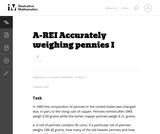Unrestricted Use
CC BY
Rating

This problem involves solving a system of algebraic equations from a context: depending how the problem is interpreted, there may be one equation or two.

Subject:
Mathematics
Algebra
Material Type:
Activity/Lab
Provider:
Illustrative Mathematics
Provider Set:
Illustrative Mathematics
Author:
Illustrative Mathematics
08/15/2012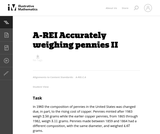Unrestricted Use
CC BY
Rating

This task is a somewhat more complicated version of "Accurately weighing pennies I'' as a third equation is needed in order to solve part (a) explicitly. Instead, students have to combine the algebraic techniques with some additional problem-solving (numerical reasoning, informed guess-and-check, etc.)

Subject:
Mathematics
Algebra
Material Type:
Activity/Lab
Provider:
Illustrative Mathematics
Provider Set:
Illustrative Mathematics
Author:
Illustrative Mathematics
08/15/2012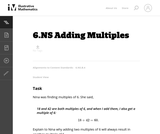Unrestricted Use
CC BY
Rating

Subject:
Mathematics
Material Type:
Activity/Lab
Provider:
Illustrative Mathematics
Provider Set:
Illustrative Mathematics
Author:
Illustrative Mathematics
05/01/2012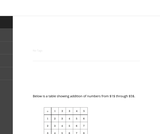Unrestricted Use
CC BY
Rating

The purpose of this task is to study some patterns in a small addition table. Each pattern identified persists for a larger table and if more time is available for this activity students should be encouraged to explore these patterns in larger tables.

Subject:
Mathematics
Numbers and Operations
Material Type:
Activity/Lab
Provider:
Illustrative Mathematics
Provider Set:
Illustrative Mathematics
Author:
Illustrative Mathematics
08/15/2012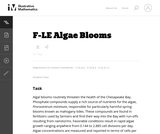Unrestricted Use
CC BY
Rating

The problem statement describes a changing algae population as reported by the Maryland Department of Natural Resources. In part (a), students are expected to build an exponential function modeling algae concentration from the description given of the relationship between concentrations in cells/ml and days of rapid growth (F-LE.2).

Subject:
Mathematics
Functions
Material Type:
Activity/Lab
Provider:
Illustrative Mathematics
Provider Set:
Illustrative Mathematics
Author:
Illustrative Mathematics
05/01/2012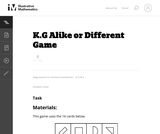Unrestricted Use
CC BY
Rating

In this game activity students practice comparing shapes and naming something that is alike or different about them.

Subject:
Mathematics
Geometry
Material Type:
Activity/Lab
Provider:
Illustrative Mathematics
Provider Set:
Illustrative Mathematics
Author:
Illustrative Mathematics
05/01/2012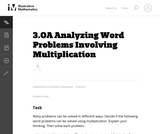Unrestricted Use
CC BY
Rating

In this task, the students are not asked to find an answer, but are asked to analyze word problems and explain their thinking. In the process, they are faced with varying ways of thinking about multiplication.

Subject:
Mathematics
Numbers and Operations
Material Type:
Activity/Lab
Provider:
Illustrative Mathematics
Provider Set:
Illustrative Mathematics
Author:
Illustrative Mathematics
05/01/2012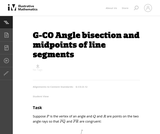Unrestricted Use
CC BY
Rating

This task provides a construction of the angle bisector of an angle by reducing it to the bisection of an angle to finding the midpoint of a line segment. It is worth observing the symmetry -- for both finding midpoints and bisecting angles, the goal is to cut an object into two equal parts. The conclusion of this task is that they are, in a sense, of exactly equivalent difficulty -- bisecting a segment allows us to bisect and angle (part a) and, conversely, bisecting an angle allows us to bisect a segment (part b). In addition to seeing how these two constructions are related, the task also provides an opportunity for students to use two different triangle congruence criteria: SSS and SAS.

Subject:
Mathematics
Geometry
Material Type:
Activity/Lab
Provider:
Illustrative Mathematics
Provider Set:
Illustrative Mathematics
Author:
Illustrative Mathematics
01/11/2013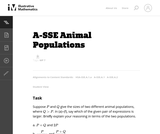Unrestricted Use
CC BY
Rating

In this task students have to interpret expressions involving two variables in the context of a real world situation. All given expressions can be interpreted as quantities that one might study when looking at two animal populations.

Subject:
Mathematics
Algebra
Material Type:
Activity/Lab
Provider:
Illustrative Mathematics
Provider Set:
Illustrative Mathematics
Author:
Illustrative Mathematics
05/01/2012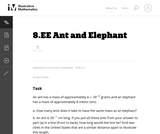Unrestricted Use
CC BY
Rating

In this problem students are comparing a very small quantity with a very large quantity using the metric system. The metric system is especially convenient when comparing measurements using scientific notations since different units within the system are related by powers of ten.

Subject:
Mathematics
Material Type:
Activity/Lab
Provider:
Illustrative Mathematics
Provider Set:
Illustrative Mathematics
Author:
Illustrative Mathematics